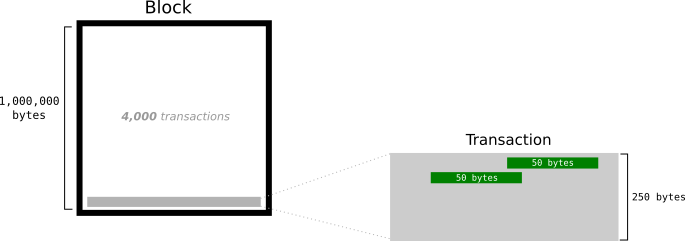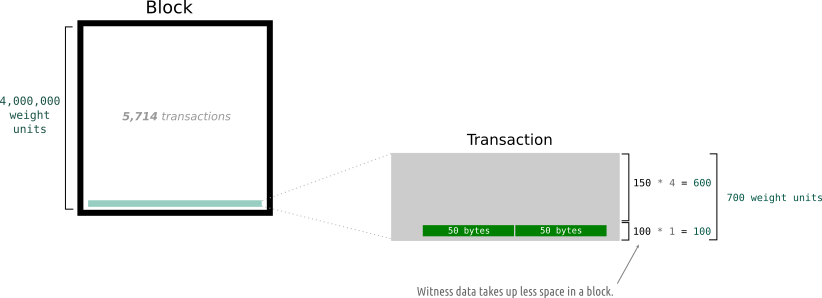# Segwit Blocksize

I want ask why Segwit enabled block can be larger than 1 MB?

“It was also intended to mitigate a blockchain size limitation problem that reduces bitcoin transaction speed

Also,

https://en.bitcoin.it/wiki/Segregated_Witness

Segregated Witness (abbreviated as SegWit) is an implemented protocol upgrade intended to provide protection from transaction malleability

SegWit was the last protocol change needed to make the Lightning Network safe to deploy on the Bitcoin network.

1 Like

Due to the fact that the unlocking code has been moved to a new “witness” field in the transaction data, this means the way block sizes are calculated can also be changed.

Currently, the block size limit is 1,000,000 bytes (1MB). Transactions are measured in bytes.With Segregated Witness, the block size limit is no longer measured in bytes . Instead, blocks and transactions are given a new metric called “weight”.

• A block has a maximum weight of `4,000,000` .
• A typical byte in a transaction weighs `4` .
• A witness byte in a transaction weighs `1` .So basically, the block size limit is multiplied by 4 to give you the new block weight limit . Each byte in a transaction is then also multiplied by 4 to give you a transaction weight . However, you only multiply the bytes of witness data by 1, which basically gives you a 75% discount on how much space it takes up in a block.

So you could say that unlocking data takes up 1/4 of the space it used to, which frees up more space in the block for transactions.

Is this a block size increase?
Yes, each block can now be greater than 1,000,000 bytes (1MB) in size.

So whilst the block size limit has not been increased to a specific number of bytes, the discounted weight for unlocking data means that blocks will typically exceed the current 1MB limit.

How much of a “block size increase” is Segregated Witness?
1.8MB, roughly.

Where did I get this figure from?
A typical block of transactions currently consists of around 60% unlocking data. 1

So if we calculate the weight of a 1MB block consisting of a “typical” amount of locking data, we get:

400,000 bytes * 4 = 1,600,000 weight units
600,000 bytes * 1 = 600,000 weight units

Total Weight = 2,200,000 weight units
Now, if a block can weigh a maximum of 4,000,000 weight units, we can work out how much of an increase this gives us:

4,000,000 / 2,200,000 = 1.81
So you could say that this is an effective block size limit increase to 1.8MB.

Just because the block size limit is changing from 1,000,000 bytes to 4,000,000 weight units, it does not make Segregated Witness an effective block size increase to 4MB, because a typical block is not going to be filled exclusively with witness data (1 weight unit per byte).

Instead, transactions will contain a combination of normal data (4 weight units) and witness data (1 weight unit). So the “block size increase” will vary depending on the composition of transactions in a block.

1 Like